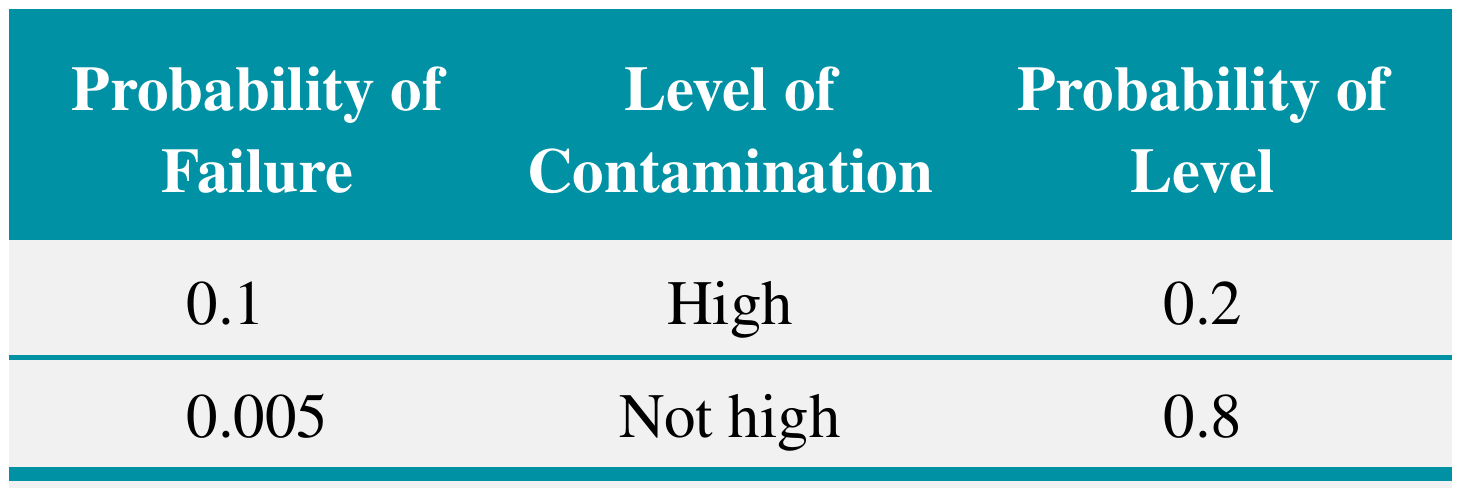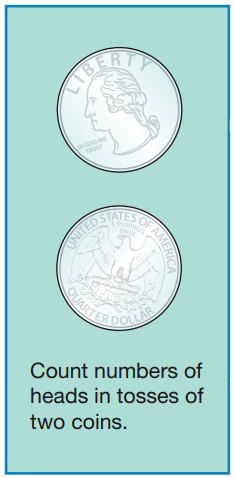# Bayes' Theorem and Probability Distributions

02/10/2021

## Instructions:

Use the left and right arrow keys to navigate the presentation forward and backward respectively. You can also use the arrows at the bottom right of the screen to navigate with a mouse.

FAIR USE ACT DISCLAIMER:
This site is for educational purposes only. This website may contain copyrighted material, the use of which has not been specifically authorized by the copyright holders. The material is made available on this website as a way to advance teaching, and copyright-protected materials are used to the extent necessary to make this class function in a distance learning environment. The Fair Use Copyright Disclaimer is under section 107 of the Copyright Act of 1976, allowance is made for “fair use” for purposes such as criticism, comment, news reporting, teaching, scholarship, education and research.

## Outline

• The following topics will be covered in this lecture:

• Bayes' theorem
• Random variables
• Probability distributions
• Probability Mass Functions

## Bayes’ Theorem

• Let us suppose that $$A$$ and $$B$$ are events for which $$P(A)\neq 0$$ and $$P(B)\neq 0$$.
• Consider the statement of the multiplication rule, $P(A \cap B) = P(A\vert B) P(B);$
• yet it is also true that, $P(B \cap A) = P(B \vert A) P(A);$
• and $$P( A \cap B) = P(B \cap A)$$ by definition.
• Putting these statements together, we obtain, \begin{align} &P(A\vert B) P(B) = P(B \vert A ) P(A)\\ \Leftrightarrow & P(A \vert B) = \frac{P(B\vert A) P(A)}{ P(B)} \end{align}
• The statement that $P(A \vert B) = \frac{P(B\vert A) P(A)}{ P(B)}$ is known as Bayes' theorem for $$P(B)>0$$.
• This is nothing more than re-writing the multiplication rule as discussed above, but the result is extremely powerful.
• Bayes' theorem wasn’t widely used in statistics for hundreds of years, until advances in digital computers.
• When digital computers became available, many tools became available using Bayes' theorem as the basis.

## Bayes' theorem continued

• Often, Bayes $P(A \vert B) = \frac{P(B\vert A) P(A)}{ P(B)}$ is used as a way to update the probability of $$A$$ when you have new information $$B$$.
• For example, let the events $$A=$$"it snows in the Sierra" and $$B=$$"it rains in my garden".
• I might think there is a $$P(A)$$ prior probability for snow, without knowing any other information.
• $$P(A\vert B)$$ is the posterior probability of snow in the Sierra given rain in my garden.
• If I found out later in the day that there was rain in my garden, I could update $$P(A)$$ to $$P(A\vert B)$$ by multiplying $P(A\vert B) = P(A) \times \left(\frac{P(B\vert A)}{P(B)}\right)$ directly.
• Although this is a simplistic example, this logic is the basis of many weather prediction techniques.

### Bayes' theorem example 1

• EXAMPLE: suppose that 20% of email messages are spam. The word free occurs in 60% of the spam messages. 13% of the overall messages contain the word free.

• Question: How can we use Bayes' theorem,

$P(A\vert B) = \frac{P(B\vert A) P(A)}{P(B)}$ to compute the probability of a message being spam, given that it includes the word “free”?

• Let the events be
• $$S=$$ “message is spam” $P(S)=0.2$
• $$F=$$ “message contains the word free” $P(F)=0.13$
• We are looking for $$P(S|F)$$
• The probability of a message that has free in it given that is spam is $P(F|S)=0.6$
• From Bayes' theorem $P(S|F)=\frac{P(F|S)P(S)}{P(F)}$
• $P(S|F)=\frac{0.6(0.2)}{0.13}=0.923$

### Bayes' theorem example 2Courtesy of Montgomery & Runger, Applied Statistics and Probability for Engineers, 7th edition

• EXAMPLE: recall the chips subject to high levels of contamination. The information is summarized in the table on the left.
• Question: How can we use Bayes' theorem, $P(A\vert B) = \frac{P(B\vert A) P(A)}{P(B)}$ to find the conditional probability of a high level of contamination present, given that a failure occurred?
• Let the events be
• $$H=$$"chip is exposed to high levels of contamination" $P(H)=0.20$
• $$F=$$"product fails"
• Earlier we computed $$P(F)$$ using the total probability rule as $P(F)=P(F|H)P(H)+P(F|H')P(H')=0.024$ with $P(F|H)=0.10 \text{ and } P(F\vert H') = 0.005$
• The probability of $$P(H | F)$$ is determined from Bayes' theorem \begin{align} P(H|F)&=\frac{P(F|H)P(H)}{P(F)} =\frac{0.10(0.20)}{0.024}=0.83\end{align}

## Random VariablesCourtesy of Mario Triola, Essentials of Statistics, 6th edition

• The first concept that we will need to develop is the random variable.
• Prototypically, we can consider the coin flipping example from the motivation:
• $$x$$ is the number heads in two coin flips.
• Every time we repeat two coin flips $$x$$ can take a different value due to many possible factors:
• how much force we apply in the flip;
• air pressure;
• wind speed;
• etc…
• The result is so sensitive to these factors that are beyond our ability to control, we consider the result to be by chance.
• Before we flip the coin twice, the value of $$x$$ has yet-to-be determined.
• After we flip the coin twice, the value of $$x$$ is fixed and possibly known.
• Formally we will define:
• Random Variable
A random variable is a function that assigns a real number to each outcome in the sample space of a random experiment.
• Notation
A random variable is denoted by an uppercase letter such as $$X$$. After an experiment is conducted, the measured value of the random variable is denoted by a lowercase letter such as $$x$$